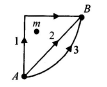Courses

# Gravitation Practice Level -1

## 25 Questions MCQ Test | Gravitation Practice Level -1

Description
This mock test of Gravitation Practice Level -1 for Class 11 helps you for every Class 11 entrance exam. This contains 25 Multiple Choice Questions for Class 11 Gravitation Practice Level -1 (mcq) to study with solutions a complete question bank. The solved questions answers in this Gravitation Practice Level -1 quiz give you a good mix of easy questions and tough questions. Class 11 students definitely take this Gravitation Practice Level -1 exercise for a better result in the exam. You can find other Gravitation Practice Level -1 extra questions, long questions & short questions for Class 11 on EduRev as well by searching above.
QUESTION: 1

Solution:
QUESTION: 2

Solution:
QUESTION: 3

### The radius of a planet is R. A satellite revolves aroundit in a circle of radius r with angular velocity ω0  . Theacceleration due to the gravity on planet’s surface is

Solution:
QUESTION: 4

A space vehicle approaching a planet has a speed v,when it is very far from the planet. At that momenttangent of its trajectory would miss the centre of theplanet by distance R. If the planet has mass M andradius r, what is the smallest value of R in order thatthe resulting orbit of the space vehicle will just missthe surface of the planet?

Solution:
QUESTION: 5

The two planets with radii R1,R2 have densities ρ1, ρ2 and atmospheric pressures p1 and p2, respectively. Therefore, the ratio of masses of their atmospheres, neglecting variation of g and p within the limits of atmosphere, is

Solution:
QUESTION: 6

If g is the acceleration due to gravity on the earth’ssurface, the change in the potential energy of an objectof mass m raised from the surface of the earth to aheight equal to the radius R of the earth is

Solution:
QUESTION: 7

A space station is set up in space at a distance equalto the earth’s radius from the surface of the earth.Suppose a satellite can be launched from the spacestation. Let v1 and v2 be the escape velocities of thesatellite on the earth’s surface and space station,respectively. Then

Solution:
QUESTION: 8

The orbital velocity of an artificial satellite in a circularorbit just above the earth’s surface is v. For a satelliteorbiting at an altitude of half of the earth’s radius, theorbital velocity is

Solution:
QUESTION: 9

The distance of two planets from the Sun are 1013 and1012 m, respectively. The ratio of time periods of thesetwo planets is

Solution:
QUESTION: 10

The gravitational force between two objects isproportional to 1/R (and not as 1/R2) where R isseparation between them, then a particle in circularorbit under such a force would have its orbital speed vproportional to

Solution:
QUESTION: 11

Two particles of equal mass go around a circle of radius R under the action of their mutual gravitational attraction. The speed of each particle is

Solution:
QUESTION: 12

A satellite of mass m is revolving around the earth atheight R radius of the earth) from the earth’s surface.Its potential energy will be

Solution:
QUESTION: 13

If g is same at a height h and at a depth d, then

Solution:
QUESTION: 14

A geostationary satellite orbits around the earth in acircular orbit of radius 36000 km. Then, the time periodof a spy satellite orbiting a few 100 km above the earth’ssurface (Rearth = 6400 km) will approximately be

Solution:
QUESTION: 15

if W1,W2and W3 represent the work done in moving a particle —from A to B along three different paths 1,2 and 3, respectively, (as shown in the figure) in the gravitational field of a point mass m, find the correct relation between W1 W2 and W3.Solution:
QUESTION: 16

If R is the radius of the earth and g the accelerationdue to gravity on the earth’s surface, the mean densityof the earth is

Solution:
QUESTION: 17

A satellite moves around the earth in a circular orbitwith speed V lf m is the mass of the satellite, its totalenergy is

Solution:
QUESTION: 18

The value of g (acceleration due to gravity) at earth’ssurface is 10 ms-2 Its value in m s-2 at the centre of theearth which is assumed to be a sphere of radius Rmetre and uniform mass density is

Solution:
QUESTION: 19

Two satellites A and B of masses m1 and m2 (m = 2 m2)are moving in circular orbits of radii r1 and r2 (r1 = 4 r2).respectively, around the earth. If their periods areTA and TB then the ratio TA / TB is

Solution:
QUESTION: 20

If three uniform spheres, each having mass M andradius R, are kept in such a way that each touches theother two, the magni-tude of the gravitational force onany sphere due to the other two is

Solution:
QUESTION: 21

If the radius of the earth decreases by 10%, the massremaining unchanged, what will happen to theacceleration due to gravity?

Solution:
QUESTION: 22

Two equal masses each m are hung from a balancewhose scale pans differ in vertical height by 'h'. Theerror in weighing in terms of density of the earth p is

Solution:
QUESTION: 23

The distances from the centre of the earth, where theweight of a body is zero and one-fourth that of the weightof the body on the surface of the earth are (assume Ris the radius of the earth)

Solution:
QUESTION: 24

Two bodies with masses M1 and M2 are initially at restand a distance R apart. Then they move directly towardsone another under the influence of their mutualgravitational attraction. What is the ratio of the distancestravelled by M1 to the distance travelled by M2?

Solution:
QUESTION: 25

If g is acceleration due to gravity on the earth’s surface,the gain the potential energy of an object of mass mraised from the surface of earth to a height equal to theradius R of the earth is

Solution: